# Relational Operations Using MapReduce

In How Map Reduce Let You Deal With PetaByte Scale With Ease, an introduction to how map reduce works and what are the reasons for it to be easily scalable were discussed. In case you are not familiar with the how map reduce works, I recommend you go through that first.

MapReduce can let us process over such high scale but, the interesting thing to know is that we can implement relational algebra operations using map reduce, which makes it possible for these systems to give an abstraction to the end users who are already familiar with SQL to just write SQL queries and get the desired results. The goal of this article is to explain how the relational operations are implemented using MapReduce jobs and visualize it for each operation using an example. This gives us an understanding on why columnar databases exist and are better for OLAP queries which needs to work by aggregation on a lot of data. Also it tells us what is the complication and complexity associated with each operation when it’s implemented using MapReduce jobs as opposed to using indices in OLTP systems which are efficient only when we make point queries. In the article the concern isn’t on how to implement the operations efficiently but to know what are the operations needed and how those operations let us maintain data independence across workers while performing those operations.

# Contents

• Relational Algebra
• Data Representation For a Table
• Hash Function
• Communication Cost
• Selections Using Map Reduce
• Projections Using Map Reduce
• Union Using Map Reduce
• Intersection Using Map Reduce
• Difference Using Map Reduce
• Grouping and Aggregation Using Map Reduce
• Conclusion

# Relational Algebra

Before getting a brief overview of relational algebra we need to know what a relation represents. As most of us are already familiar with SQL there is no point on putting a long description here. A relation represents a database table. A major point that distinguishes SQL and relational algebra is that in relational algebra duplicate rows are implicitly eliminated which is not the case with SQL implementations. Here in this article implementation of relational algebra operations is discussed, but it’s easily generalizable to the implementations that don’t eliminate duplicates. If a reader is familiar with relational algebra they can just skim over this section.

• Selection: selection(WHERE clause in SQL) lets you apply a condition over the data you have and only get the rows that satisfy the condition.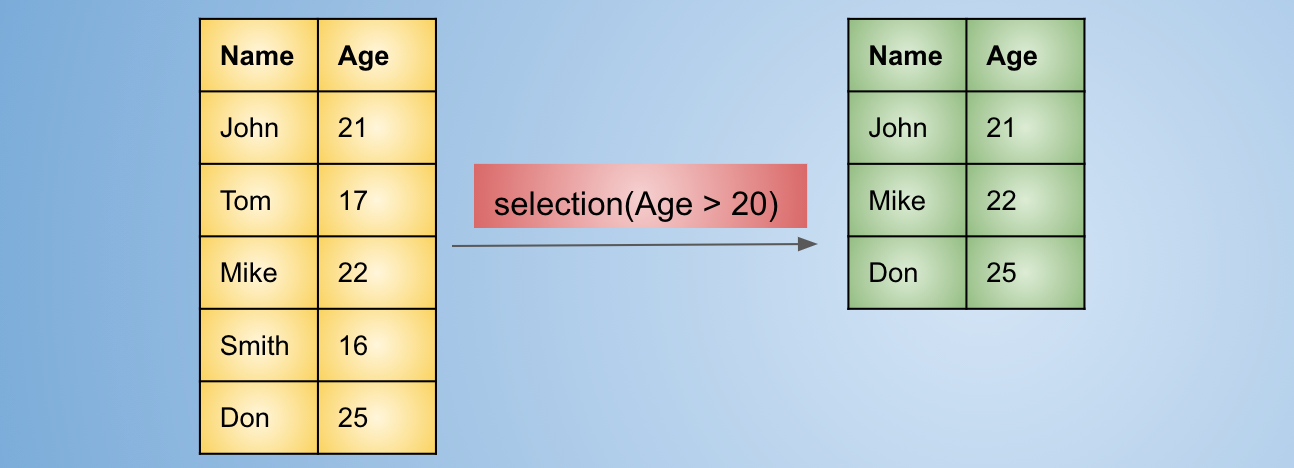Selection operation to select only rows where age is greater than 20
• Projection: In order to select some columns only we use the projection operator. It’s analogous to SELECT in SQL.
• Union: We concatenate two tables vertically. Similar to UNION in SQL, but the duplicate rows are removed implicitly. The point to note in the below output table is that `(Smith, 16)`was a duplicate row so it appears only once in the output where as `(Tom, 17)` , `(Tom, 19)` appears as two, as those are not identical rows.
• Intersection: Same as INTERSECT in SQL. It intersects two tables and selects only the common rows.
• Difference: The rows that are in the first table but not in second are selected for output. Keep in mind that `(Monty, 21)` is not considered in the output as its present in the second table but not in first.
• Natural Join: Merge two tables based on some common column. It represents the INNER JOIN in SQL. But the condition is implicit on the column that is common in both tables. The output will only contain rows for which the values in the common column matches. It will generate one row for every time the column value across two tables match as is the case below for `Tom` . If there were multiple `Tom` values in the table on the top in the image below, then four rows would have been created in the output table representing all the combinations.
• Grouping and Aggregation: Group rows based on some set of columns and apply some aggregation (sum, count, max, min, etc.) on some column of the small groups that are formed. This corresponds to GROUP BY in SQL.

# Data Representation For a Table

In a distributed storage system the entire table isn’t stored on a single node(computer), the most relevant reason being it doesn’t fit completely on a single system because of its large size. So in order to store a table the table is partitioned into small files which are distributed across the nodes available in the system. Why some abstraction of partitions is a must for distributed storage HDFS docs is a good place to goto.

Let’s have a simple abstraction on how data is stored in the system. Think of tables as being stored as small CSV files which if concatenated will represent the table.

# Hash Function

Hash function can be something like

`1. Take a key2. Typecast it to string3. For each character in the string sum up the ASCII value4. Mod the sum with number of reduce workers, this value is the hash value for that particular key.`

You can check in link in resources section how people compute hashes of strings. We just want a hash function in this case that will distribute the work equally among reduce workers. Even if we have high number of collisions it’s fine as we are not using hash function to construct maps which allows fast search, we just want data to be partitioned in `n` buckets where `n` is the number of reduce workers while making sure data for same key goes to the same reduce worker across all worker nodes.

# Communication Cost

As is the case with all the systems, the performance of a system is measured on the bases of the least efficient component of that system. In Map Reduce system that component is the network and the cost associated with this component is termed as communication cost. The communication cost of a task is the number of rows input to that task. Here task represents either a map task or reduce task. We consider number of rows as a measure of size as mostly the data is in tabular form having a predefined schema, because of which size will almost be the same for each row. So the amount of data sent over the network is proportional to the number of rows. The sum of all the communication costs associated with all the tasks is the communication cost of the operation.

We don’t consider output of a task for computing this cost as output will be an input to the next task, so this will end up getting counted twice.

# Selection Using Map Reduce

To perform selections using map reduce we need the following Map and Reduce functions:

• Map Function: For each row `r` in the table apply condition and produce a key value pair `r, r` if condition is satisfied else produce nothing. i.e. key and value are the same.
• Reduce Function: The reduce function has nothing to do in this case. It will simply write the value for each key it receives to the output.

For our example we will do`Selection(B <= 3)`. Select all the rows where value of B is less than or equal to 3.

Let’s consider the data we have initially distributed as files in Map Workers, And the data looks like the following figure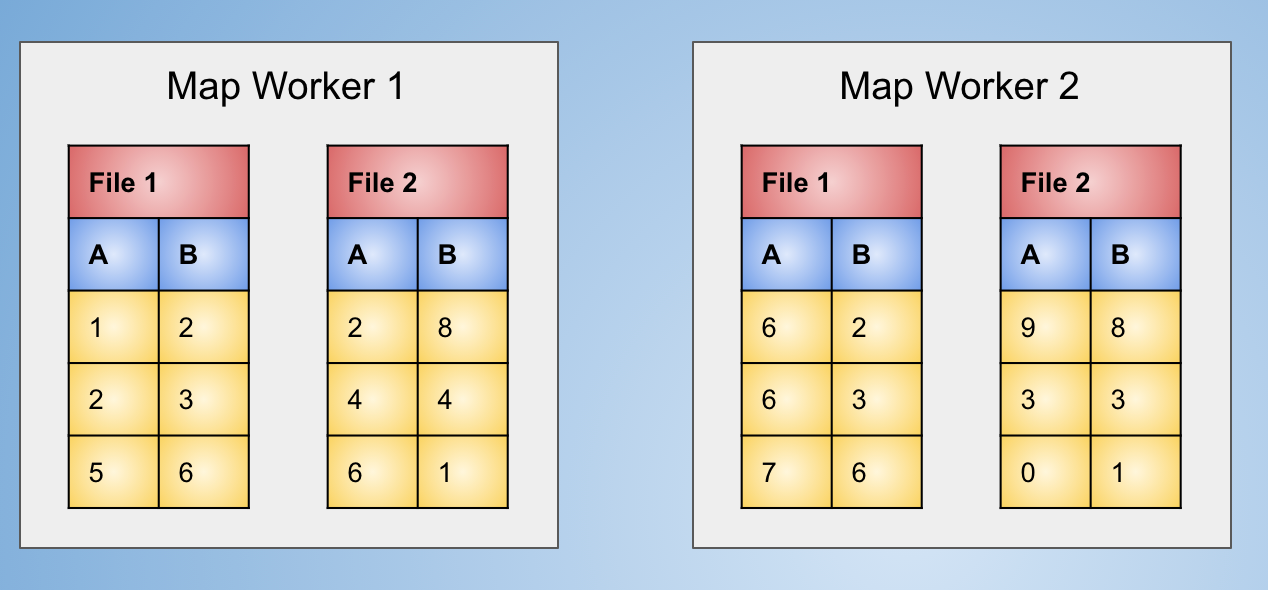Initial data distributed in files across map workers representing a single table

After applying the map function (And grouping, there are no common keys in this case as each row is unique) we will get the output as follows, The tuples are constructed with 0th index containing values from A column and 1st index containing values from B. In actual implementations either this information can be sent as some extra metadata or within each value itself, making values and keys look something like `({A: 1}, {B: 2})`, which does look somewhat inefficient.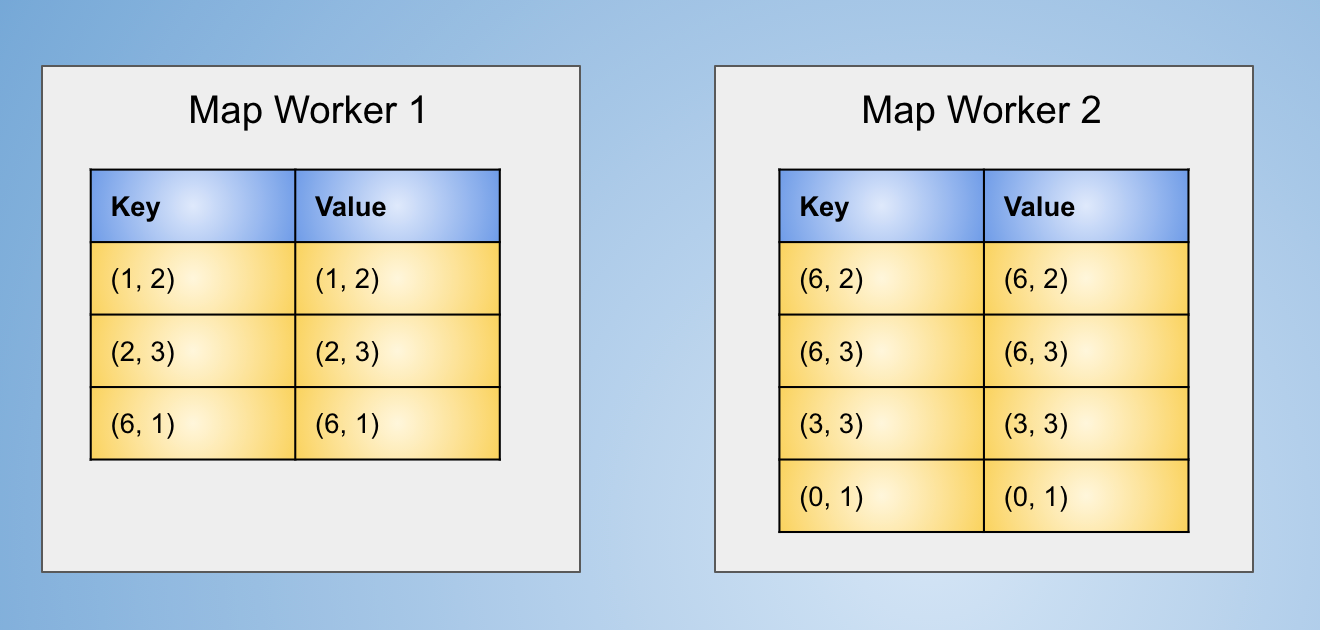Data after applying Map function which filtered rows having B value less than 3

After this based on number or reduce workers (2 in our case). A hash function is applied as explained in the Hash Function section. The files for reduce workers on map workers will look like: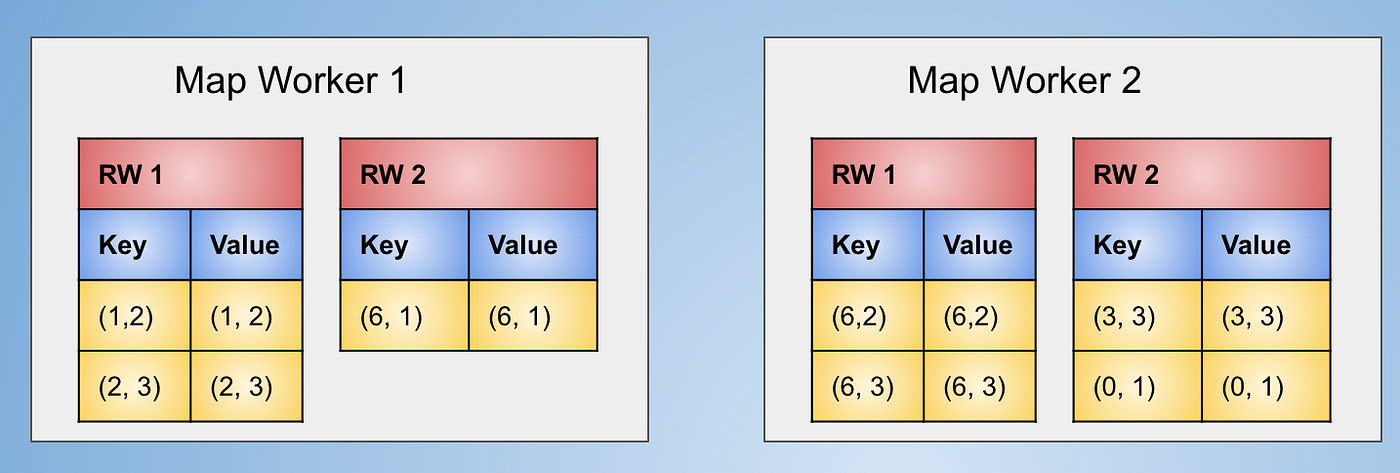Files for reduce workers created at map worker based on hash function

After this step The files for reduce worker 1 are sent to that and reduce worker 2 are sent to that. The data at reduce workers will look like:

The final output after applying the reduce function which ignores the keys and just consider values will look like:

The points to take into consideration here are that we don’t need to shuffle data across the nodes really. We can just execute the map function and save values to the output from map workers itself. This makes it an efficient operation (When compared to others where reduce function does something).

# Projection Using Map Reduce

• Map Function: For each row `r` in the table produce a key value pair `r', r’`, where `r'` only contains the columns which are wanted in the projection.
• Reduce Function: The reduce function will get outputs in the form of `r' :[r', r', r', r', ...]`. As after removing some columns the output may contain duplicate rows. So it will just take the value at 0th index, getting rid of duplicates (Note that this de duplication is done as we are implementing the operations while getting outputs which we are supposed to get according to relational algebra).

Let’s see it in action, by computing `projection(A, B)` for the following table:

After application of map function (ignoring values in C column) and grouping the keys the data will look like:

The keys will be partitioned using a hash function as was the case in selection. The data will look like:

The data at the reduce workers will be:

At the reduce node the keys will be aggregated again as same keys might have occurred at multiple map workers. As we already know the reduce function operates on values of each key only once.

The reduce function is applied which will consider only the first value of the values list and ignore rest of the information.

The points to remember are that here the reduce function is required for duplicate elimination. If that’s not the case (as it is in SQL) We can get rid of reduce operation, meaning we don’t have to move data around. So, this operation can be implemented without actually passing data around.

# Union Using Map Reduce

Both selection and projection are operations that are applied on a single table, whereas Union, intersection and difference are among the operations that are applied on two or more tables. Let’s consider that schemas of the two tables are the same, and columns are also ordered in same order.

• Map Function: For each row `r` generate key-value pair `(r, r)` .
• Reduce Function: With each key there can be one or two values (As we don’t have duplicate rows), in either case just output first value.

This operations has the map function of the selection and reduce function of projection. Let’s see the working using an example. Here yellow colour represents one table and green colour is used to represent the other one stored at two map workers.

After applying the map function and grouping the keys we will get output as:

The data to be sent to reduce workers will look like:

Data at reduce workers after will be:

At reduce workers aggregation on keys will be done.

The final output after applying the reduce function which takes only the first value and ignores everything else is as follows:

Here we note that in this case same as projection we can this done without moving data around in case we are not interested in removing duplicates. And hence this operation is also efficient it terms of data shuffle across machines.

# Intersection Using Map Reduce

For intersection, let’s consider the same data we considered for union and just change the map and reduce functions

• Map Function: For each row `r` generate key-value pair `(r, r)` (Same as union).
• Reduce Function: With each key there can be one or two values (As we don’t have duplicate rows), in case we have length of list as 2 we output first value else we output nothing.

As the map function is same as union and we are considering the same data lets skip to the part before reduce function is applied.

Now we just apply the reduce operation which will output only rows if list has a length of 2.

# Difference Using Map Reduce

Let’s again consider the same data. The difficulty with difference arises with the fact that we want to output a row only if it exists in the first table but not the second one. So the reduce function needs to keep track on which tuple belongs to which relation. To visualize that easier we will keep those rows green which come from 2nd table and yellow for which come from 1st table and purple which comes from both tables.

• Map Function: For each row `r` create a key-value pair `(r, T1)` if row is from table 1 else product key-value pair `(r, T2)`.
• Reduce Function: Output the row if and only if the value in the list is `T1` , otherwise output nothing.

The data taken initially is the same as it was for union

After applying the map function and grouping the keys the data looks like the following figure

After applying map function files for reduce workers will be created based on hashing keys as has been the case so far.

The data at the reduce workers will look like

After aggregation of the keys at reduce workers the data looks like:

The final output is generated after applying the reduce function over the output.

For the difference operation we notice that we cannot get rid of the reduce part and hence have to send data across the workers as the context of from which table the value came is needed. Hence it will be more expensive operation as compared to selection, projection, union and intersection.

# Grouping and Aggregation Using Map Reduce

Usually understanding grouping and aggregation takes a bit of time when we learn SQL, but not in case when we understand these operations using map reduce. The logic is already there in the working of the map. Map workers implicitly group keys and the reduce function acts upon the aggregated values to generate output.

• Map Function: For each row in the table, take the attributes using which grouping is to be done as the key, and value will be the ones on which aggregation is to be performed. For example, If a relation has 4 columns A, B, C, D and we want to group by A, B and do an aggregation on C we will make (A, B) as the key and C as the value.
• Reduce Function: Apply the aggregation operation (sum, max, min, avg, …) on the list of values and output the result.

For our example lets group by `(A, B)` and apply `sum` as the aggregation.

The data after application of map function and grouping keys will creates `(A, B)` as key and `C` as value and `D` is discarded as if it doesn’t exist.

Applying partitioning using hash functions, we get

The data at the reduce workers will look like

The data is aggregated based on keys before applying the aggregation function (sum in this case).

After applying the sum over the value lists we get the final output

Here also like difference operation we can’t get rid of the reduce stage. The context of tables isn’t wanted here but the aggregation function makes it necessary for the values to be in one place for a single key. This operation is also inefficient as compared to selection, projection, union, and intersection. The column that is not in aggregation or grouping clause is ignored and isn’t required. So if the data be stored in a columnar format we can save cost of loading a lot of data. Usually there are only a few columns involved in grouping and aggregation it does save up a lot of cost both in terms of data that is sent over the network and the data that needs to be loaded to main memory for execution.

The natural join will keep the rows that matches the values in the common column for both tables. To perform natural join we will have to keep track of from which table the value came from. If the values for the same key are from different tables we need to form pairs of those values along with key to get a single row of the output. Join can explode the number of rows as we have to form each and every possible combination of the values for both tables.

• Map Function: For two relations `Table 1(A, B)` and `Table 2(B, C)` the map function will create key-value pairs of form `b: [(T1, a)]` for table 1 where T1 represents the fact that the value `a` came from table 1, for table 2 key-value pairs will be of the form `b: [(T2, c)]`.
• Reduce Function: For a given key `b` construct all possible combinations for the values where one value is from table `T1` and the other value is from table `T2`. The output will consist of key-value pairs of form `b: [(a, c)]` which represent one row `a, b, c` for the output table.

For an example lets consider joining `Table 1` and `Table 2`, where `B` is the common column.

The data after applying the map function and grouping at the map workers will look like: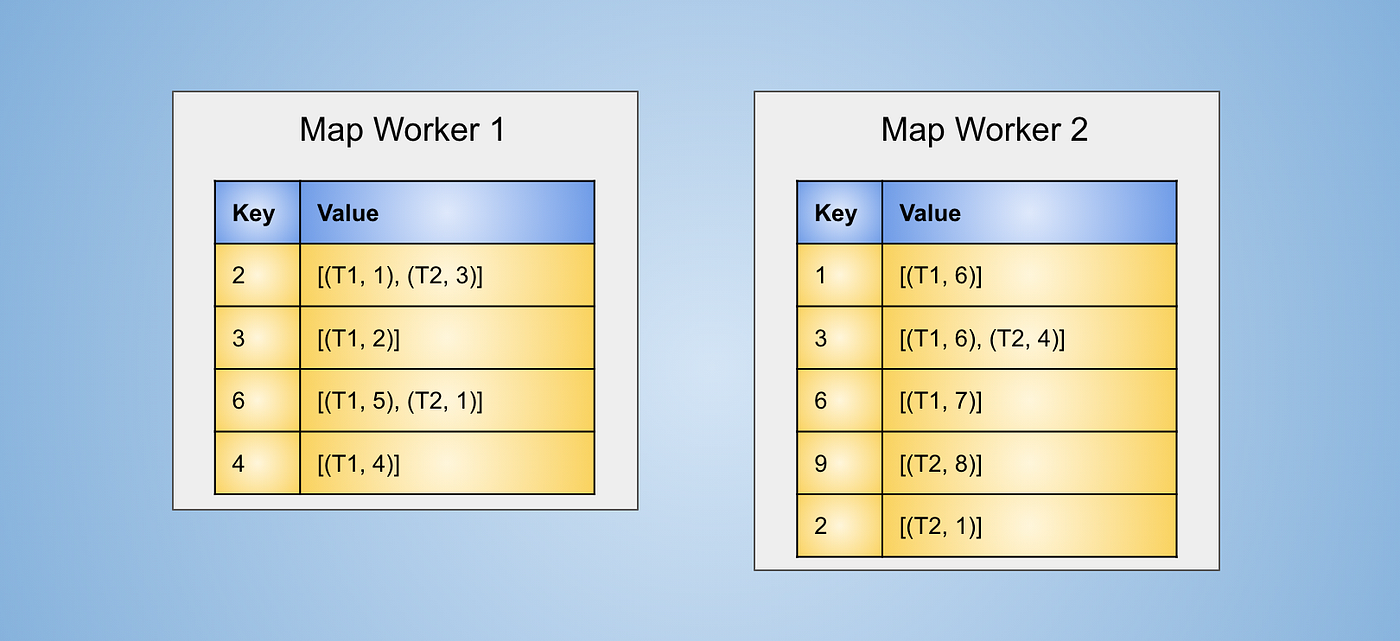Data at map workers after applying map function and grouping the keys

As has been the case so far files for reduce workers will be created at the map workers

The data at the reduce workers will be:

Applying aggregation of keys at the reduce workers we get:

After applying the reduce function which will create a row by taking one value from table T1 and other one from T2. If there are only values from T1 or T2 in the values list that won’t constitute a row in output.

As we need to keep context from which table a value came from, we can’t get rid of the data that needs to be sent across the workers for application of reduce task, this operation also becomes costly as compared to others we discussed so far. The fact that for each list of values we need to create pairs also plays a major factor in the computation cost associated with this operation.

# Conclusion

The operations discussed in the article constitute the most regularly used operations while performing analysis or transformation over the data. We can see that almost all the operations which output less number of columns as compared to the number of columns in the parent table will benefit from having columnar storage as those files can be skipped from being transferred to tasks which will lower the communication cost.

The point to keep in mind is that using the operations as specified in this article how we were able to keep data at one worker independent from the data at the other workers, making it possible for the system to have high level of parallelism. The operations as can be seen can be made more efficient, in some cases we can decrease the communication cost to just reading data from disk to memory (disks might not be as slower as networks but still are slower enough to be considered in communication cost, if not considered we can even get zero communication cost).

--

--